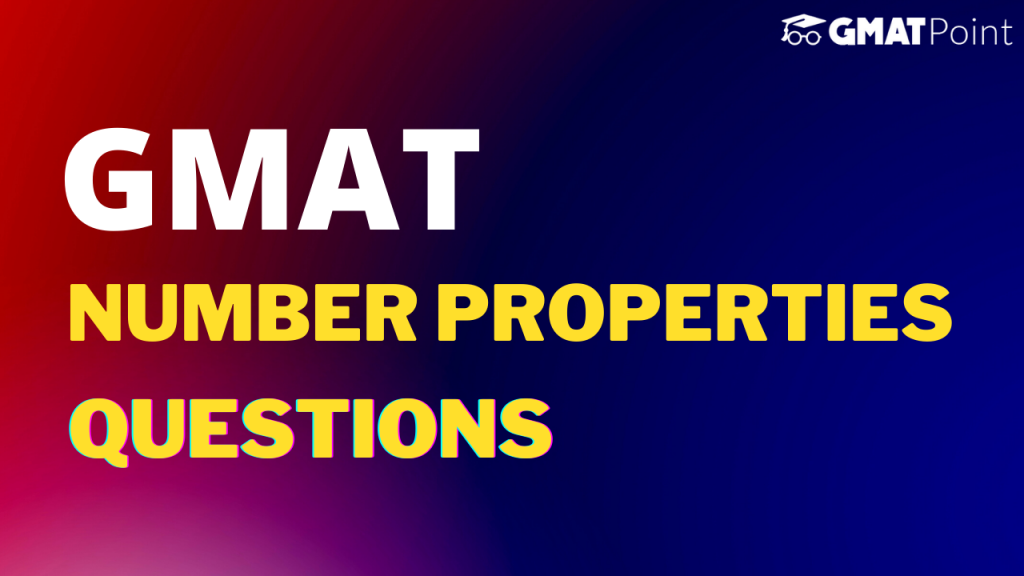# GMAT Number Properties: Pratice Questions# GMAT Number Properties Questions

Number Properties questions constitute a major portion of the Quant section of the GMAT. These questions can come under both Problem Solving and Data Sufficiency types. In this article, we will be looking into –

1. GMAT Number Properties Questions with Answers

## Examples – GMAT Number Properties Questions with Answers and explanations

### Question 1:

Which of the following numbers is odd? a = even b = odd

a) ab + a + b + 1

b) a + b – ab – 1

c) a + b – 1

d) 3b + 2a

e) 4a – 6b

### Approach:

ab = even x odd = even

a) even + even + odd + odd = even

b) even + odd – even – odd = even

c) even + odd – odd = even

d) odd + even = odd

e) even + even = even

Hence, Option D is odd.

### Question 2:

The largest two-digit number having an odd number of factors is?

### Approach:

Odd number of factors -> Number is a perfect square.

Hence, 81.

### Question 3:

If two numbers are co-rime, and both of them are greater than 1, find out the highest possible difference between these numbers, given that both are less than 100.

### Approach:

The smallest possible number is 2. The largest possible number is 99.

They are co-prime.

99 – 2 = 97.

### Note:

Though these examples provide a good sense of what type of GMAT Number Properties questions you can expect, in no way do they represent the exhaustive list of concepts required for the Quantitative section of the GMAT.

### Tips to keep in mind:

1. Try to take some time out from the Problem-Solving Number Properties questions so that you can use that time to solve the tricky Data Sufficiency Number Properties questions.
2. Do not get stuck in a question for long. If you find yourself trapped in a question for long, take a guess and move on.
3. Look out for negation words. For example: Which of the following are NOT possible values of x?
4. Some questions can be solved faster by the use of options. Make sure you don’t solve these questions in a conventional way.

You can check out the Free GMAT Daily Targets on our platform.

Also, check out the Free GMAT Verbal Tests and Quant Tests.

If you are starting your GMAT preparation from scratch, do check out GMATPOINT.